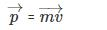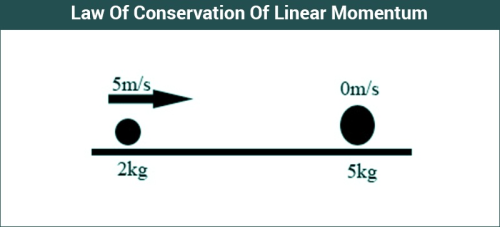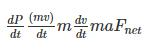Courses

# Law of Conservation of Linear Momentum Class 11 Notes | EduRev

## Class 11 : Law of Conservation of Linear Momentum Class 11 Notes | EduRev

The document Law of Conservation of Linear Momentum Class 11 Notes | EduRev is a part of Class 11 category.
All you need of Class 11 at this link: Class 11

Needed a Document for problems on law of conservation of linear momentum for bsc students?

Ref: https://edurev.in/question/696783/Needed-a-Document-for-problems-on-law-of-conservat

The linear momentum of a particle at an instant is defined as the product of its mass and velocity at that particular instant. It is represented by the symbol p, and it is a vector quantity.mv

The momentum of a system of particles is the vector sum of the individual momentum of all the particles.

## Law of Conservation of Linear Momentum:

If the net external force acting on a system of bodies is zero, then the momentum of the system remains constant. This is the basic law of conservation of linear momentum.

We have to remember that the momentum of the system is conserved and not that of the individual particles. The momentum of the individual bodies in the system might increase or decrease according to the situation, but the momentum of the system will always be conserved, as long as there is no external net force acting on it.### Explanation:

The law of conservation of momentum can be explained from the second law of motion. Newton’s second law of motion says that rate of change of linear momentum of a body is equal to the net external force applied on it.

Mathematically it is expressed as:Fnet

If the net external force acting on a body is zero, then the rate of change of momentum is also zero, which means that there is no change in momentum.

#### Example:

Two bodies of mass M and m are moving in opposite directions with the velocities v. If they collide and move together after the collision, we have to find the velocity of the system.

Since there is no external force acting on the system of two bodies, momentum will be conserved.

Initial momentum = Final momentum

(Mv – mv) = (M+m)VFinal

From this equation, we can easily find the final velocity of the system.

#### Applications:

One of the applications of conservation of momentum is the launching of rockets. The rocket fuel burns are pushed the exhaust gases downwards, and due to this, the rocket gets pushed upwards. Motorboats also work on the same principle, it pushes the water backward, and gets pushed forwards in reaction to conserve momentum.

Offer running on EduRev: Apply code STAYHOME200 to get INR 200 off on our premium plan EduRev Infinity!

,

,

,

,

,

,

,

,

,

,

,

,

,

,

,

,

,

,

,

,

,

;